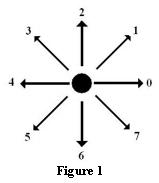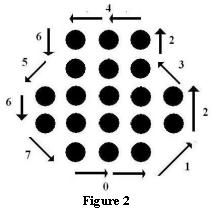Problems

# Chain Code

Time limit 1 second
Memory limit 64 MiB

In a black and white (bi-level) image, collections of connected black pixels can be defined as foreground or objects, while white can be thought of as background. Each set of connected black pixels can be completely described by listing the positions of the pixels on its boundary in counterclockwise order, starting at some arbitrary point on the boundary. This list of pixels can, in turn, be represented simply as the direction to the next one in the list. This list of directions is called the chain code of the object, and describes the shape of the object precisely while being position independent.There are 8 possible directions from one pixel to an adjacent pixel, and while assigning these numbers is arbitrary, figure 1 shows the standard convention. The direction 0 means "to the right of", 2 "means immediately above", and 1 is at 45 degrees, bisecting 0 and 2, and so on.

Two black pixels are considered to be adjacent if the square of the distance between them is less than or equal to 2. This is based on a standard graphics coordinate system having a pixel at each integer coordinate. Two pixels are connected if a contiguous path of adjacent pixels can be traced between them. A connected region is a set of black pixels in which all members are connected to each other. A boundary pixel of a connected region (from now on just a region) is a pixel within the region that has at least one neighbor (in the four compass directions) that is not black. For this problem, you may assume that there are no "holes" in the region, so that there is only one boundary of the region.

The chain code of a region can start at any pixel on the boundary. It proceeds by finding the next adjacent pixel on the boundary in a counter-clockwise direction, saving the direction (0-7) in an output buffer, and then continuing the process from the new pixel. When we arrive at the starting pixel again, the chain code is complete. The output buffer contains a set of direction values which comprise the chain code itself, and from which the original set of pixels can be recreated starting at any pixel position in an image.As an example, a chain code for the region in figure2 is 446567001232. The chain code describes the shape of the region unambiguously, although its position is completely unknown. Shape related measures such as perimeter and area (number of pixels in the region) can be determined directly from the chain code description alone. You are to write a program that calculates the area of a connected region given only the chain code.

## Input data

The input will be a collection of chain code strings, one per line. Each chain code contains at most 1000000 characters. You may assume that each chain code describes a valid region, and does not describe a boundary that intersects itself.

## Output data

For each chain code in the input, the output will be the area of the region (i.e. the number of pixels belonging to it), each printed on its own line.

## Examples

Input example #1
446567001232
6024

Output example #1
19
4